# Please show all work for both I need to be able to reproduce the work with...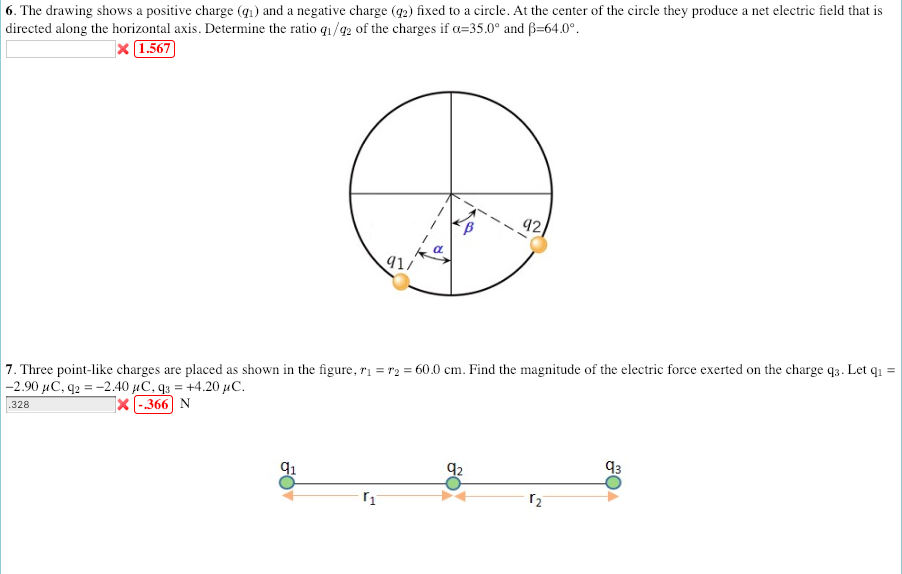Please show all work for both I need to be able to reproduce the work with different numbers. Thank you

6. The drawing shows a positive charge () and a negative charge (2) fixed to a circle. At the center of the circle they produce a net electric field that is directed along the horizontal axis. Determine the ratio q1/42 of the charges if α-350° and β-640°. X 1.567 1/ 7. Three point-like charges are placed as shown in the figure, ri r2 = 60.0 cm. Find the magnitude of the electric force exerted on the charge q3-Let qi 328 X366 N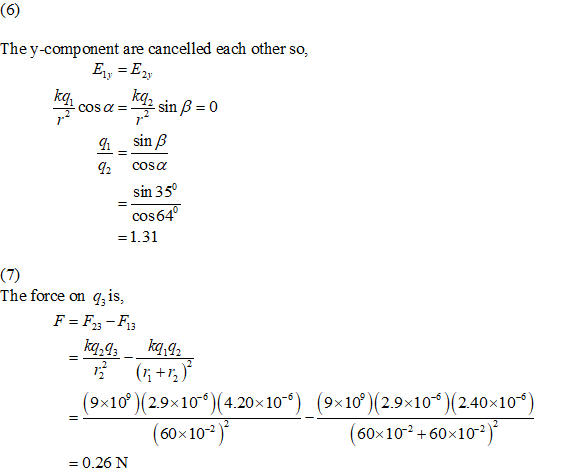##### Add Answer of: Please show all work for both I need to be able to reproduce the work with...
Similar Homework Help Questions
• ### The drawing shows a positive charge (q1q1) and a positive charge (q2q2) fixed to a circle....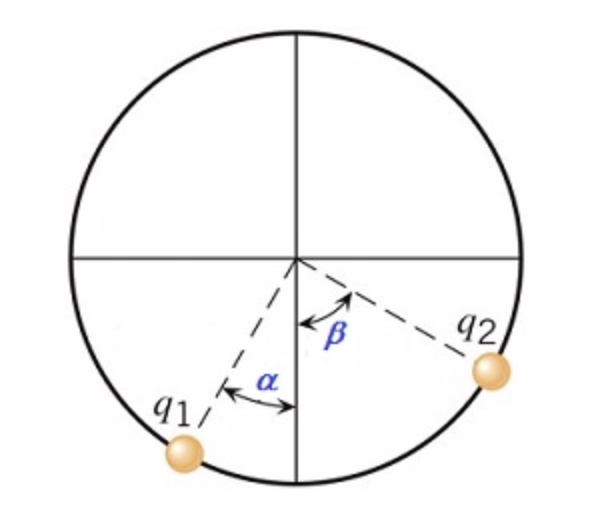The drawing shows a positive charge (q1q1) and a positive charge (q2q2) fixed to a circle. At the center of the circle they produce a net electric field that is directed along the vertical axis. Determine the ratio q1/q2 q1/q2 of the charges if α=26.0° and β=60.0°. 42 ra q1/

• ### The drawing shows a negative charge (q1) and a negative charge (q2) fixed to a circle....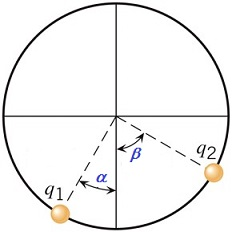The drawing shows a negative charge (q1) and a negative charge (q2) fixed to a circle. At the center of the circle they produce a net electric field that is directed along the vertical axis. Determine the ratio q1/q2 of the charges if α=29.0° and β=56.0°. 42

• ### 6. The drawing shows a positive charge (qı) and a negative charge (g2) fixed to a...6. The drawing shows a positive charge (qı) and a negative charge (g2) fixed to a circle. At the center of the circle they produce a net electric field that is directed along the horizontal axis. Determine the ratio q1/92 of the charges if α=33.0° and β=560。 x1.52218 Show my other submissions 42 1/

• ### 6. The drawing shows a positive charge (i) and a positive charge (ga) fixed to a...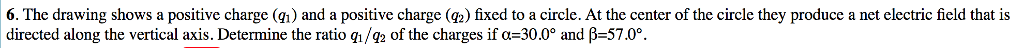6. The drawing shows a positive charge (i) and a positive charge (ga) fixed to a circle. At the center of the circle they produce a net electric field that is directed along the vertical axis. Determine the ratio q/Q2 of the charges if α-300° and β=57.0°. q1/

• ### Please Help me Immediately. I only have 3 hours to submit!!! 7. Three point-like charges are...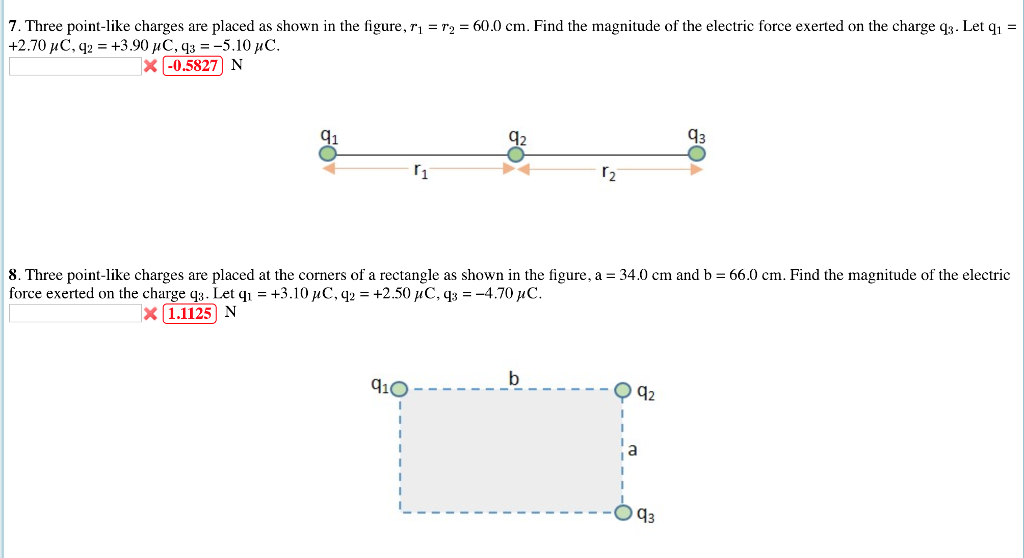Please Help me Immediately. I only have 3 hours to submit!!! 7. Three point-like charges are placed as shown in the figure, r2 60.0 cm. Find the magnitude of the electric force exerted on the charge qa. Let q1 -0.5827N 41 T1 r2 8. Three point-like charges are placed at the corners of a rectangle as shown in the figure, a = 34.0 cm and b force exerted on the charge q3. Let qi-+ 66.0 cm Find the magnitude of...

• ### The drawing shows a positive charge (q1q1) and a positive charge (q2q2) fixed to a circle....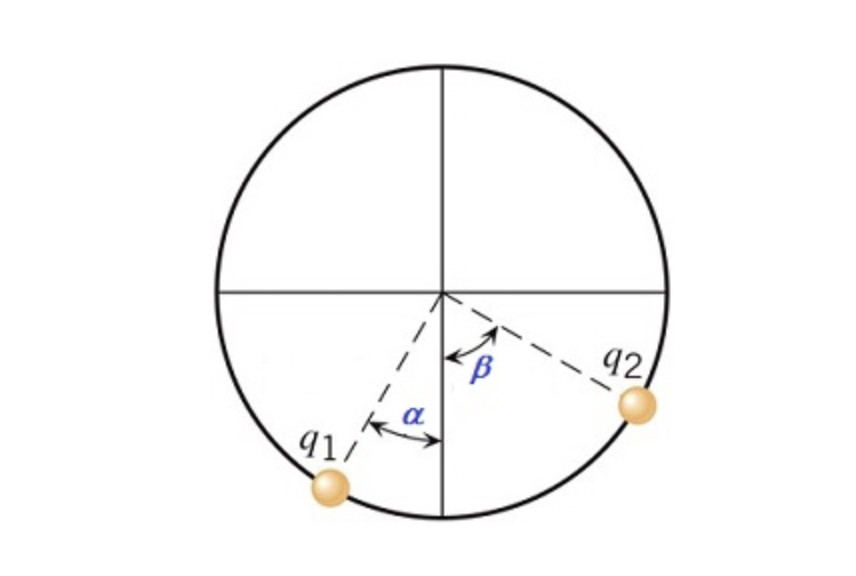The drawing shows a positive charge (q1q1) and a positive charge (q2q2) fixed to a circle. At the center of the circle they produce a net electric field that is directed along the vertical axis. Determine the ratio q1/q2q1/q2 of the charges if α=27.0° and β=57.0°.

• ### 6. The drawing shows a negative charge (a) and a negative charge (g2) fixed to a...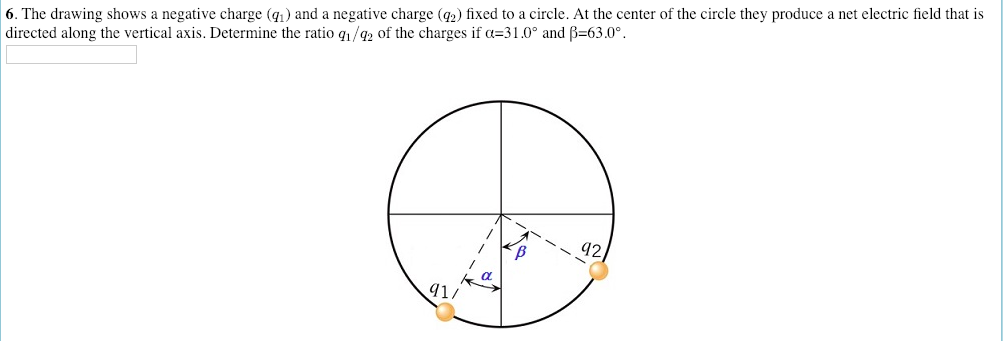6. The drawing shows a negative charge (a) and a negative charge (g2) fixed to a circle. At the center of the circle they produce a net electric field that is directed along the vertical axis. Determine the ratio ql/Q Of the charges if α-31 0° and β=63.0。 42 1/

• ### 6. The drawing shows a positive charge (g) and a negative charge (g2) fixed to a...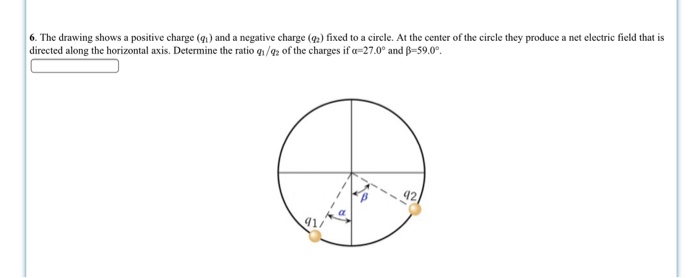6. The drawing shows a positive charge (g) and a negative charge (g2) fixed to a circle. At the center of the circle they produce a net electric field that is directed along the horizontal axis. Determine the ratio g1/02 of the charges if α-27.0° and β-59.0° 42 41/

• ### 6. The drawing shows a negative charge (q) and a positive charge (q2) fixed to a...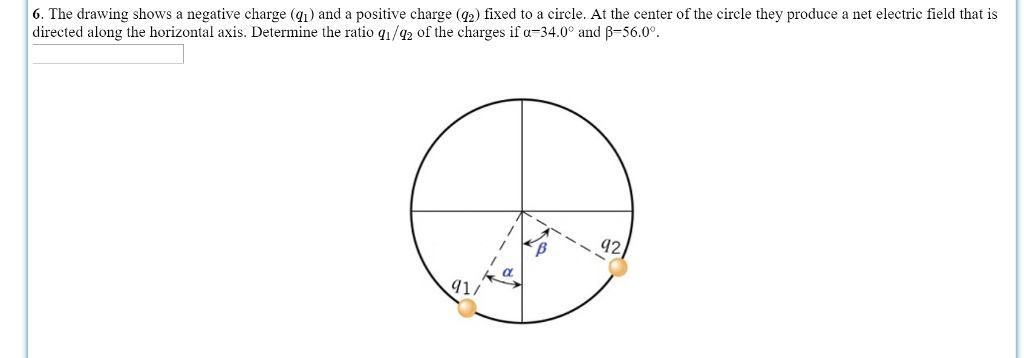6. The drawing shows a negative charge (q) and a positive charge (q2) fixed to a circle. At the center of the circle they produce a net electric field that is directed along the horizontal axis. Determine the ratio q1/42 of the charges if α 34.0 and -56.0° 41/

• ### Please see question below. Please double check all work and units/unit conversions. Show all work. Thank...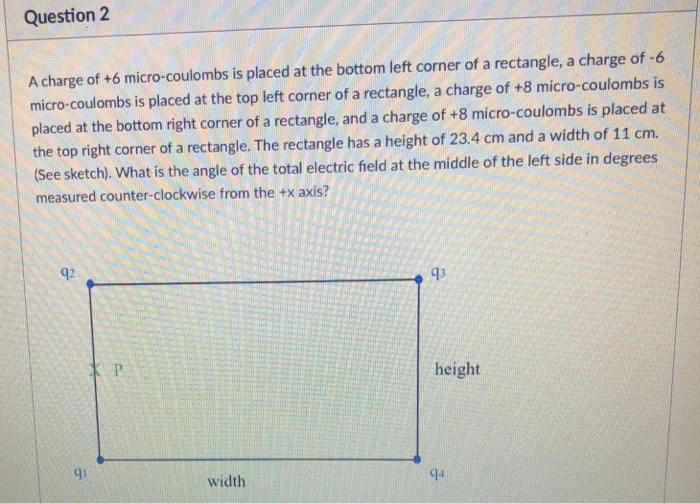Please see question below. Please double check all work and units/unit conversions. Show all work. Thank you! Question 2 A charge of +6 micro-coulombs is placed at the bottom left corner of a rectangle, a charge of -6 micro-coulombs is placed at the top left corner of a rectangle, a charge of +8 micro-coulombs is placed at the bottom right corner of a rectangle, and a charge of +8 micro-coulombs is placed at the top right corner of a rectangle....

Need Online Homework Help?